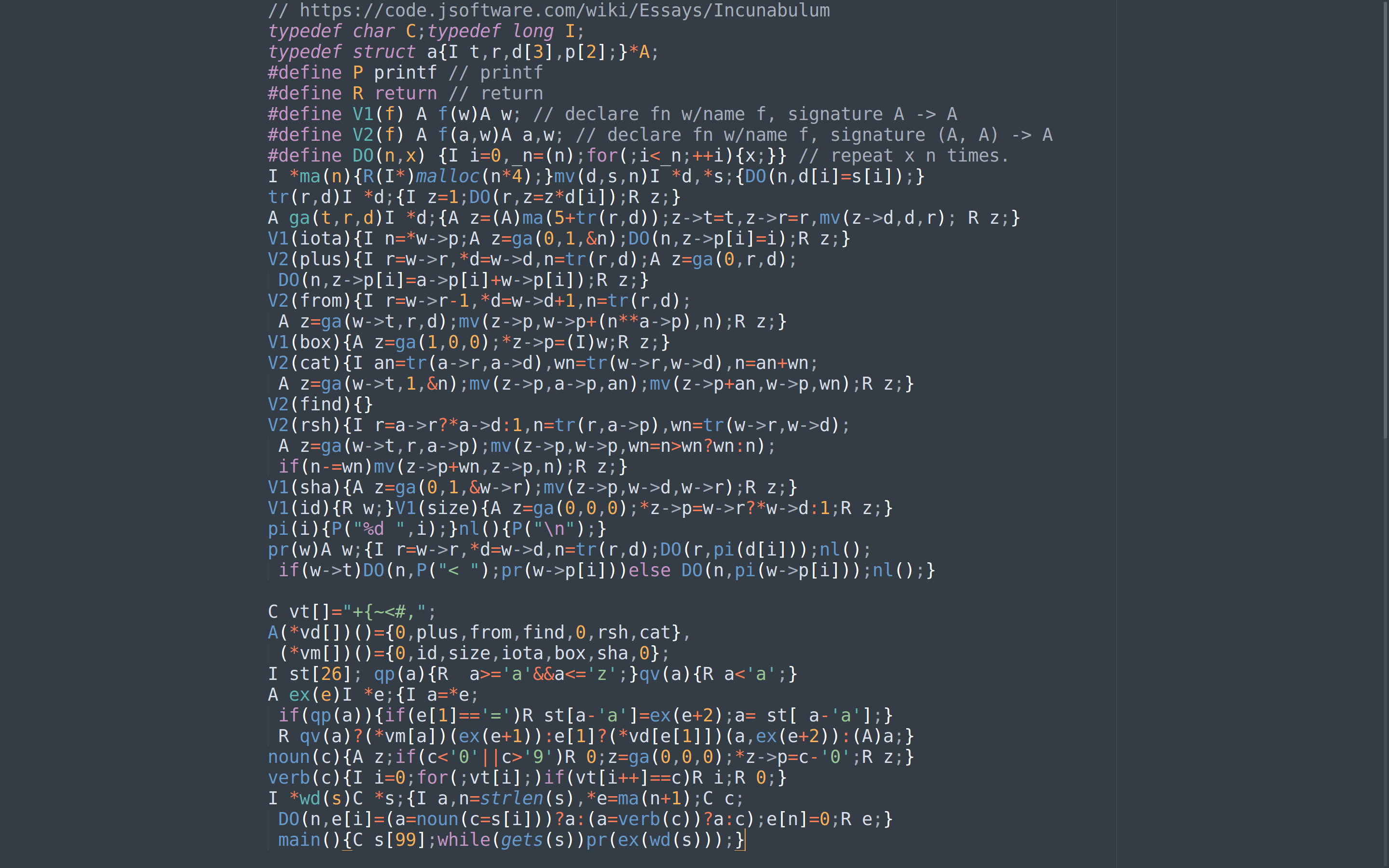## § Incunabulum for the 21st century: Making the J interpreter compile in 2020

This is me trying to understand the fabled interpreter of the J language working, so I could absorb Arthur Whitney's style of writing C: it's cryptic, short, and fits in a page. I learnt of this from the J language page , which comes with the quote:
One summer weekend in 1989, Arthur Whitney visited Ken Iverson at Kiln Farm and produced—on one page and in one afternoon—an interpreter fragment on the AT&T 3B1 computer. I studied this interpreter for about a week for its organization and programming style; and on Sunday, August 27, 1989, at about four o'clock in the afternoon, wrote the first line of code that became the implementation described in this document. Arthur's one-page interpreter fragment is as follows:

#### § The original source

typedef char C;typedef long I;
typedef struct a{I t,r,d,p;}*A;
#define P printf
#define R return
#define V1(f) A f(w)A w;
#define V2(f) A f(a,w)A a,w;
#define DO(n,x) {I i=0,_n=(n);for(;i<_n;++i){x;}}
I *ma(n){R(I*)malloc(n*4);}mv(d,s,n)I *d,*s;{DO(n,d[i]=s[i]);}
tr(r,d)I *d;{I z=1;DO(r,z=z*d[i]);R z;}
A ga(t,r,d)I *d;{A z=(A)ma(5+tr(r,d));z->t=t,z->r=r,mv(z->d,d,r);
R z;}
V1(iota){I n=*w->p;A z=ga(0,1,&n);DO(n,z->p[i]=i);R z;}
V2(plus){I r=w->r,*d=w->d,n=tr(r,d);A z=ga(0,r,d);
DO(n,z->p[i]=a->p[i]+w->p[i]);R z;}
V2(from){I r=w->r-1,*d=w->d+1,n=tr(r,d);
A z=ga(w->t,r,d);mv(z->p,w->p+(n**a->p),n);R z;}
V1(box){A z=ga(1,0,0);*z->p=(I)w;R z;}
V2(cat){I an=tr(a->r,a->d),wn=tr(w->r,w->d),n=an+wn;
A z=ga(w->t,1,&n);mv(z->p,a->p,an);mv(z->p+an,w->p,wn);R z;}
V2(find){}
V2(rsh){I r=a->r?*a->d:1,n=tr(r,a->p),wn=tr(w->r,w->d);
A z=ga(w->t,r,a->p);mv(z->p,w->p,wn=n>wn?wn:n);
if(n-=wn)mv(z->p+wn,z->p,n);R z;}
V1(sha){A z=ga(0,1,&w->r);mv(z->p,w->d,w->r);R z;}
V1(id){R w;}V1(size){A z=ga(0,0,0);*z->p=w->r?*w->d:1;R z;}
pi(i){P("%d ",i);}nl(){P("\n");}
pr(w)A w;{I r=w->r,*d=w->d,n=tr(r,d);DO(r,pi(d[i]));nl();
if(w->t)DO(n,P("< ");pr(w->p[i]))else DO(n,pi(w->p[i]));nl();}

C vt[]="+{~<#,";
A(*vd[])()={0,plus,from,find,0,rsh,cat},
(*vm[])()={0,id,size,iota,box,sha,0};
I st; qp(a){R  a>='a'&&a<='z';}qv(a){R a<'a';}
A ex(e)I *e;{I a=*e;
if(qp(a)){if(e=='=')R st[a-'a']=ex(e+2);a= st[ a-'a'];}
R qv(a)?(*vm[a])(ex(e+1)):e?(*vd[e])(a,ex(e+2)):(A)a;}
noun(c){A z;if(c<'0'||c>'9')R 0;z=ga(0,0,0);*z->p=c-'0';R z;}
verb(c){I i=0;for(;vt[i];)if(vt[i++]==c)R i;R 0;}
I *wd(s)C *s;{I a,n=strlen(s),*e=ma(n+1);C c;
DO(n,e[i]=(a=noun(c=s[i]))?a:(a=verb(c))?a:c);e[n]=0;R e;}

main(){C s;while(gets(s))pr(ex(wd(s)));}

It's a lot to take in --- it's quite breathtaking really, the way it all hangs together in one page.

#### § The attempt to get it run

Unfortunately, this does not work if we try to get it to run in 2020. I decided to read the code and understand what would happen. I managed to read enough to understand that the code a=~3 ought to create an array with values [0 1 2]. On attempting to run this however, we get:
\$ gcc -O0 -g -std=c89 -fsanitize=address -fsanitize=undefined incunabulum.c -o bin/incunabulum && ./bin/incunabulum
...
(many many GCC warnings elided)
...
a=~3
=================================================================
0x60300000eff0 at pc 0x000000402be3 bp 0x7ffe6dde6b70 sp 0x7ffe6dde6b60
WRITE of size 8 at 0x60300000eff0 thread T0
#0 0x402be2 in wd /home/bollu/work/w/incunabulum.c:40
#1 0x402d28 in main /home/bollu/work/w/incunabulum.c:42
#2 0x7f7ae901082f in __libc_start_main (/lib/x86_64-linux-gnu/libc.so.6+0x2082f)
#3 0x400ca8 in _start (/home/bollu/w/bin/incunabulum+0x400ca8)
...
...
==23726==ABORTING

oops! The code uses a lot of punning between int and int*. These assumptions break now that we're in 64-bit. The patch to get this to work is:

#### § the patch

diff --git a/incunabulum.c b/incunabulum.c
index 2cae744..778e35a 100644
--- a/incunabulum.c
+++ b/incunabulum.c
@@ -1,11 +1,11 @@
-typedef char C;typedef long I;
+typedef char C;typedef long long I;
typedef struct a{I t,r,d,p;}*A;
#define P printf
#define R return
#define V1(f) A f(w)A w;
#define V2(f) A f(a,w)A a,w;
#define DO(n,x) {I i=0,_n=(n);for(;i<_n;++i){x;}}
-I *ma(n){R(I*)malloc(n*4);}mv(d,s,n)I *d,*s;{DO(n,d[i]=s[i]);}
+I *ma(n){R(I*)malloc(n*8);}mv(d,s,n)I *d,*s;{DO(n,d[i]=s[i]);}
tr(r,d)I *d;{I z=1;DO(r,z=z*d[i]);R z;}
A ga(t,r,d)I *d;{A z=(A)ma(5+tr(r,d));z->t=t,z->r=r,mv(z->d,d,r);
R z;}
@@ -34,9 +34,10 @@ I st; qp(a){R  a>='a'&&a<='z';}qv(a){R a<'a';}
A ex(e)I *e;{I a=*e;
if(qp(a)){if(e=='=')R st[a-'a']=ex(e+2);a= st[ a-'a'];}
R qv(a)?(*vm[a])(ex(e+1)):e?(*vd[e])(a,ex(e+2)):(A)a;}
-noun(c){A z;if(c<'0'||c>'9')R 0;z=ga(0,0,0);*z->p=c-'0';R z;}
-verb(c){I i=0;for(;vt[i];)if(vt[i++]==c)R i;R 0;}
+I noun(c){A z;if(c<'0'||c>'9')R 0;z=ga(0,0,0);*z->p=c-'0';R z;}
+I verb(c){I i=0;for(;vt[i];)if(vt[i++]==c)R i;R 0;}
I *wd(s)C *s;{I a,n=strlen(s),*e=ma(n+1);C c;
DO(n,e[i]=(a=noun(c=s[i]))?a:(a=verb(c))?a:c);e[n]=0;R e;}

main(){C s;while(gets(s))pr(ex(wd(s)));}
+


#### § It runs!

After applying the patch, we manage to get the interpreter to run!
./bin/incunabulum
a=~3
3
0 1 2


#### § The lock screen

I liked it so much that I took a screenshot and made it my lock screen.#### § Thoughts

I'm really fascinated by the code. I loved I could simply stare the screenshot to absorb the code. There was no scrolling involved. The variables are well-named (to the extent I understand the code), and it's clearly extremely well thought out. If there's someone who understands some of the thorny aspects of the code:
• What is the t variable really tracking?
• How does one create a multi-dimensional array easily?
• What are some interesting programs one can run with this mini-interpreter?
I'd be really glad to know the details. Please leave an issue or a pull request against the repo . I'm going write a dissection of the code once I fully understand it, since I couldn't find explanaing the code on the internet. Until then, enjoy the monolith of code!